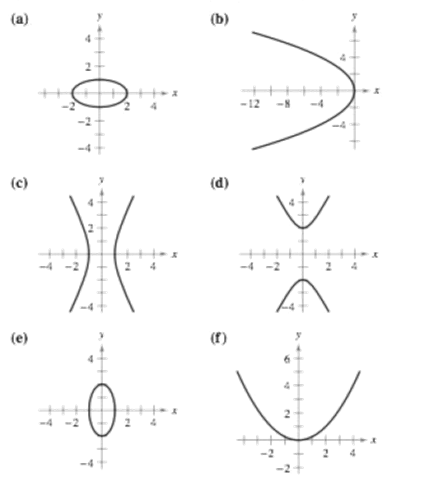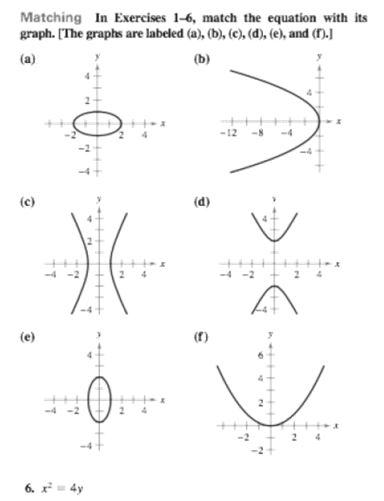Chapter 10, Problem 3RE

Chapter
Section
Textbook Problem

Matching In Exercises 1-6, match the equation with its graph. [The graphs are labeled (a), (b), (c), (d), (e), and (f).] y 2 = − 4 xTo determine
If the equation y2=4x matches with its graph when the options are:Explanation

Given:

The options are:

Explanation:

Consider the given equation y2=4x.

Here, only y has a squared term and x2 is not there. Thus, this equation represents a parabola.

In order to find whether this parabola opens horizontally or vertically, reoresent this equation in the form of standard parabola equation.

Write the equation in the standard form as:

4(x0)=(y0)2

Compare this with the standard equation of

Still sussing out bartleby?

Check out a sample textbook solution.

See a sample solution

The Solution to Your Study Problems

Bartleby provides explanations to thousands of textbook problems written by our experts, many with advanced degrees!

Get Started

Calculate y'. 39. y = tan2(sin )

Calculus: Early Transcendentals

Convert the expressions in Exercises 6584 to power form. 3

Finite Mathematics and Applied Calculus (MindTap Course List)

Perform the indicated operation for the following. 8.

Contemporary Mathematics for Business & Consumers

In Exercises 110, find the graphical solution to each inequality. xy0

Finite Mathematics for the Managerial, Life, and Social Sciences

The general solution to dydt=ky is: a) y(t) = y(0)ekt b) y(t) = y(k)et c) y(t) = y(t)ek d) y(t) = ey(0)kt

Study Guide for Stewart's Single Variable Calculus: Early Transcendentals, 8th